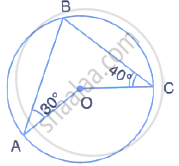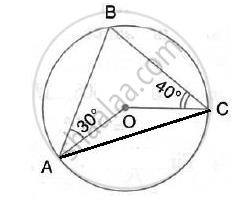Share

In the Given Figure, O is the Centre of the Circle. ∠Oab And ∠Ocb Are 30° and 40° Respectively. Find ∠Aoc . Show Your Steps of Working. - ICSE Class 10 - Mathematics

ConceptArc and Chord Properties - Angles in the Same Segment of a Circle Are Equal (Without Proof)

Question

In the given figure, O is the centre of the circle. ∠OAB and ∠OCB are 30° and 40°
respectively. Find  ∠AOC . Show your steps of working.SolutionJoin AC,
Let ∠OAC = ∠OCA = x (say)
∴ ∠AOC =180° -2x
Also, ∠BAC = 30°+ x

In ΔABC,
∠ABC =180°- ∠BAC -∠BCA
=180° - (30°+x) - (40°+ x ) = 110°- 2x
Now, ∠AOC = ∠2 ABC

(Angle at the centre is double the angle at the circumference subtended by the same chord)
⇒ 180°- 2x = 2(110° - 2x)
⇒  2x = 40
∴ x = 20
∴ ∠AOC = 180° - 2×20° = 140°

Is there an error in this question or solution?
Solution In the Given Figure, O is the Centre of the Circle. ∠Oab And ∠Ocb Are 30° and 40° Respectively. Find ∠Aoc . Show Your Steps of Working. Concept: Arc and Chord Properties - Angles in the Same Segment of a Circle Are Equal (Without Proof).
S